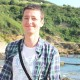# Differences Between Butterworth and Chebyshev approximations.

Hi, at my this post i will share my experiment that i learned at my digital
signal processing lessons and laboratory.
It is about filters and i am going to show you that is how to desing discrete-time filters with  Butterworth and Chebyshev approximations. Then we will use  and see the differences.Lowpass Filter

First step; we are investigating the input-output relations a filter and taking Fast Fourier Transform (FFT) of a signal.

We have the properties of a low-pass filter like following.
(Later on i will show how we did this )

0.8 < |H(exp(jw))| < 1   for     0 < w < 0.22*pi
|H(exp(jw))| < 0.26     for   0.32*pi < w < pi

This means our filter has bandpass region between 0-0.22*pi and tolereable
magnitude values 0.8 and 1 (at ideal low-pass filters our magnitude was 1)
,transition region 0.22 to 0.32 and at the end it has stopband region
0.32*pi to pi and magtinude is smaller than 0.26 (at ideal low-pass filters
our magnitude was 1). Also we will normalize this values.

```%x is the input signal
%y is the filtered output signal

f=linspace(-1,1,length(x));figure;
subplot(4,1,1);plot(x);
title('My input signal');
subplot(4,1,2);plot(y);
title('My output signal');
subplot(4,1,3);stem(f,fftshift(abs(fft(x))));
title('Magnitude response of my input signal');
subplot(4,1,4);stem(f,fftshift(abs(fft(y))));
title('Magnitude response of my output signal'););

stem(X,Y) fuction plots a signal X versus Y
linspace(a,b,n) funtion creates a row vector between a and b, divides linearly with n.ta
stem(f,fftshift(abs(fft(x)))); takes the FFT of x and
```As you see just symetric peaks. Three of them are at right side at another side. Filter’s cutoff frequency was 0.32 and just outside of 0.4 (and -0.4) is supressed so the signal at 0.6 ( and -0.6 ).
Additionally; if you want to use matlab’s help file, just type help and subject to the command window and press enter.
Like help stem. It suggests you the titles or you may know the fuction but wanna see the details ; use doc coomad like,
doc. stem .

Good Luck,
Gökhan Öztürk## ozturkgokhan hakkında

Electrical and electronics engineer. In turkey.

Aralık 3, 2015 tarihinde Maltab içinde yayınlandı ve , , , , , , olarak etiketlendi. Kalıcı bağlantıyı yer imlerinize ekleyin. Yorum yapın.